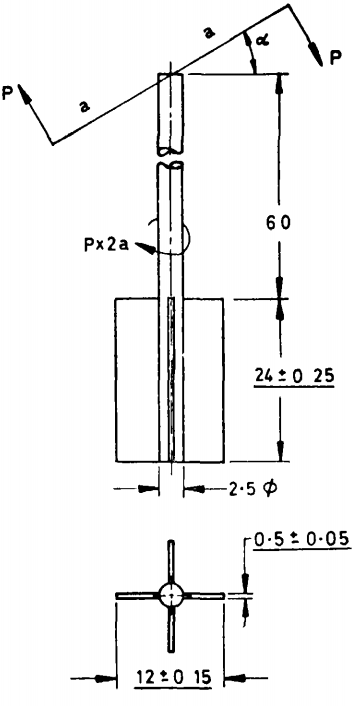# Vane Shear Test CalculatorIS 2720 (Part 30)

##Vanes Shear Calculation

kg/cm2

Test-I
degree
degree
cm
cm
Test-II
degree
degree
cm
cm
Test-III
degree
degree
cm
cm

#### Avg. Shear Strength

Cohesion

Avg. Cohesion (Cu)

$=\frac{\mathrm{Cu1\left(TestI\right)+Cu2\left(TestII\right)+Cu3\left(TestII\right)}}{3}$

$=\frac{\mathrm{0.0034+0.0069+0.0103}}{3}$

$={\mathrm{0.0069}}^{}$

#### Result of Test-I

Shear Strenghth

Undrained Shear Strenghth of the Soil

$Torque\left(T\right)=K×\frac{\mathrm{Diffrence In Degree}}{\mathrm{180}}$

$T=4×\frac{\mathrm{30}}{\mathrm{180}}={\mathrm{0.67}}^{}$

$Cu=\mathrm{0.0034}\mathrm{Kg}/{\mathrm{cm}}^{2}$

#### Result of Test-II

Shear Strenghth

Undrained Shear Strenghth of the Soil

$Torque\left(T\right)=K×\frac{\mathrm{Diffrence In Degree}}{\mathrm{180}}$

$T=4×\frac{\mathrm{60}}{\mathrm{180}}={\mathrm{1.33}}^{}$

$Cu=\mathrm{0.0069}\mathrm{Kg}/{\mathrm{cm}}^{2}$

#### Result of Test-III

Shear Strenghth

Undrained Shear Strenghth of the Soil

$Torque\left(T\right)=K×\frac{\mathrm{Diffrence In Degree}}{\mathrm{180}}$

$T=4×\frac{\mathrm{90}}{\mathrm{180}}={\mathrm{2.00}}^{}$

$Cu=\mathrm{0.0103}\mathrm{Kg}/{\mathrm{cm}}^{2}$

## What is Vane Shear Test?

vane shear test for the measurement of shear strength of cohesive soils is useful for soils of low shear strength of less than about 0'5 kgf/cml . This test gives the undrained strength of the soil and the undisturbed and rernoulded strengths obtained are used for evaluating the sensitivity of the soil.

##### Apparatus
• The apparatus may be either of the hand-operated type or motorized. Provisions should be made in the apparatus for the following:
• a) Fixing of vane and shaft to the apparatus in such a way that the vane can be lowered gradually and vertically into the soil specimen.
• b) Fixing the tube containing the soil specimen to the base of the equipment for which it should have suitable hole.
• c) Arrangement for lowering the vane into the soil specimen (contained in the tube fixed to the base) gradually and vertically and for holding the vane properly and securely in the lowered position.
• d) Arrangement for rotating the vane steadily at a rate of approximately 1/60 rev/min (O'lo/s) and for measuring the rotation of the vane.
• e) A torque applicator to rotate the vane in the soil and a device for measuring the torque applied to an accuracy of 0'05 cm.kgf.
• f) A set of springs capable of measuring shear strength of 0'5 kgf/cml .
• Vane - The vane shall consist of four blades each fixed at 90° . The vane should not deform under the maximum torque for which it is designed. The penetrating edge of the vane blades shall be sharpened having an included angle of 90°. The vane blades shall be welded together suitably to a central rod, the maximum diameter of which should preferably not exceed 25 mm in the portion of the rod which goes into the specimen during the test. The vane should be pr operly treated to prevent rusting and corrosion.Principle of Vane Shear Test

##### Procedure
• The specimen in the tube should be at least 37'5 mm in diameter and 75 mm long. Mount the specimen container with the specimen on the base of the vane shear apparatus and fix it securely to the base. If the specimen container is closed at one end it should be provided at the bottom with a hole of about I mm diameter. Lower the shear vanes into the specimen to their full length gradually with minimum disturbance of the soil specimen so that the top of the vane is at least 10 mm below the top of the specimen. Note the readings of the strain and torque indicators. Rotate the vane at a uniform rate approximately 0'1 o/s by suitably operating the torque applicator handle until the specimen fails. Note the final reading of the torque indicator. Torque readings and the corresponding strain readings may also be noted at desired intervals of time as the test proceeds.

• Just after the determination of the maximum torque rotate the vane rapidly through a minimum of ten revolutions. The remoulded strength should then be determined within I minute after completion of the revolution.Laboratory Vane Shear Apparatus

##### Vane Shear Test Calculations
$Torque\left(T\right)=K×\frac{\mathrm{Diffrence of Reading}}{\mathrm{180}}$

Where,